1. /
2. CBSE
3. /
4. Class 09
5. /
6. Triangles class 9 Notes...

# Triangles class 9 Notes Mathematics### myCBSEguide App

Download the app to get CBSE Sample Papers 2023-24, NCERT Solutions (Revised), Most Important Questions, Previous Year Question Bank, Mock Tests, and Detailed Notes.

CBSE class 9 Mathematics Chapter 7 Triangles notes in PDF are available for free download in myCBSEguide mobile app. The best app for CBSE students now provides Triangles class 9 Notes latest chapter wise notes for quick preparation of CBSE exams and school based annual examinations. Class 9 Mathematics notes on Chapter 7 Triangles are also available for download in CBSE Guide website.

## CBSE Guide Triangles class 9 Notes

CBSE guide notes are the comprehensive notes which covers the latest syllabus of CBSE and NCERT. It includes all the topics given in NCERT class 9 Mathematics text book. Users can download CBSE guide quick revision notes from myCBSEguide mobile app and my CBSE guide website.

## 9 Mathematics notes Chapter 7 Triangles

Download CBSE class 9th revision notes for Chapter 7 Triangles in PDF format for free. Download revision notes for Triangles class 9 Notes and score high in exams. These are the Triangles class 9 Notes prepared by team of expert teachers. The revision notes help you revise the whole chapter in minutes. Revising notes in exam days is on of the best tips recommended by teachers during exam days.

CBSE Class 09 Mathematics
Revision Notes
CHAPTER – 7
TRIANGLES

1. Congruence of Triangles
2. Criteria for Congruence of Triangles
3. Some Properties of a Triangle
4. Inequalities in a Triangle
• Triangle – A closed figure formed by three intersecting lines is called a triangle. A triangle has three sides, three angles and three vertices.
• Congruent figures – Congruent means equal in all respects or figures whose shapes and sizes both are  same. For example, two circles of the same radii are congruent. Also two squares of the same sides are congruent.
• Congruent Triangles – Two triangles are congruent if and only if one of them can be made to superimpose on the other, so as to cover it completely
• If two triangles ABC and PQR are congruent under the correspondence  then symbolically, it is expressed as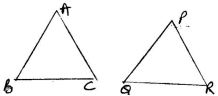• In congruent triangles, corresponding parts are equal and we write ‘CPCT’ for corresponding parts of congruent triangles.
• SAS congruency rule – Two triangles are congruent if two sides and the included angle  between two sides of one triangle are equal to the two sides and the included angle between two sides  of the other triangle. For example  and  as shown in the figure satisfy SAS congruence criterion.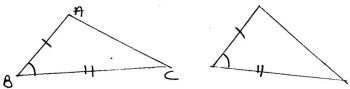• ASA Congruence Rule – Two triangles are congruent if two angles and the included side of one triangle are equal to two angles and the included side of other triangle. For examples  shown below satisfy ASA congruence criterion.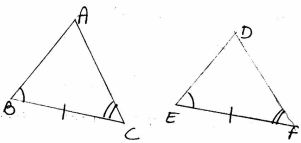• AAS Congruence Rule – Two triangle are congruent if any two pairs of angles and one pair of corresponding sides are equal.For example  shown below satisfy AAS congruence criterion.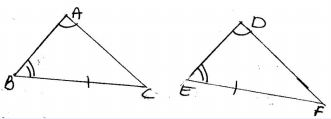• AAS criterion for congruence of triangles is a particular case of ASA criterion.
• Isosceles Triangle – A triangle in which two sides are equal is called an isosceles triangle. For example  shown below is an isosceles triangle with AB=AC.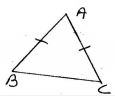• Scalene Triangle – A triangle, no two of whose sides are equal, is called scalene triangle.
• Equilateral Triangle – A triangle whose all sides are equal, is calle an equilateral triangle.
• Right angled triangle – A triangle with one right angle is called a right angled triangle.
• The sum of all the angles of a triangle is 180o.
• If a side of a triangle is produced, the exterior angle so formed is equal to the sum of two interior opposite angles.
• Angle opposite to equal sides of a triangle are equal.
• Sides opposite to equal angles of a triangle are equal.
• Each angle of an equilateral triangle is .
• If the altitude from one vertex of a triangle bisects the base, then the triangle is isosceles triangle.
• SSS congruence Rule – If three sides of one triangle are equal to the three sides of another triangle then the two triangles are congruent for example  as shown in the figure satisfy SSS congruence criterion.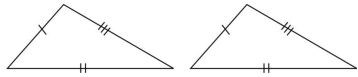• RHS Congruence Rule – If in two right triangles the hypotenuse and one side of one triangle are equal to the hypotenuse and one side of the other triangle then the two triangle are congruent. For example  shown below satisfy RHS congruence criterion.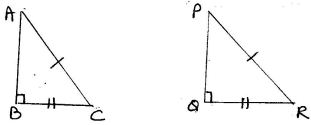RHS stands for Right angle – Hypotenuse side.
• A point equidistant from two given points lies on the perpendicular bisector of the line segment joining the two points and vice-versa.
• A point equidistant from two intersecting lines lies on the bisectors of the angles formed by the two lines.
• In a triangle, angle opposite to the longer side is larger (greater)
• In a triangle, side opposite to the larger (greater) angle is longer.
• Sum of any two sides of a triangle is greater than the third side.

## Triangles class 9 Notes

• CBSE Revision notes for Class 9 Mathematics PDF
• CBSE Revision notes Class 9 Mathematics – CBSE
• CBSE Revisions notes and Key Points Class 9 Mathematics
• Summary of the NCERT books all chapters in Mathematics class 9
• Short notes for CBSE class 9th Mathematics
• Key notes and chapter summary of Mathematics class 9
• Quick revision notes for CBSE exams

## CBSE Class-9 Revision Notes and Key Points

Triangles class 9 Notes. CBSE quick revision note for Class-9 Mathematics, Chemistry, Maths, Biology and other subject are very helpful to revise the whole syllabus during exam days. The revision notes covers all important formulas and concepts given in the chapter. Even if you wish to have an overview of a chapter, quick revision notes are here to do if for you. These notes will certainly save your time during stressful exam days.

To download Triangles class 9 Notes, sample paper for class 9 Mathematics, Social Science, Science, English Communicative; do check myCBSEguide app or website. myCBSEguide provides sample papers with solution, test papers for chapter-wise practice, NCERT solutions, NCERT Exemplar solutions, quick revision notes for ready reference, CBSE guess papers and CBSE important question papers. Sample Paper all are made available through the best app for CBSE students and myCBSEguide website.### Test Generator

Create question paper PDF and online tests with your own name & logo in minutes.### myCBSEguide

Question Bank, Mock Tests, Exam Papers, NCERT Solutions, Sample Papers, Notes